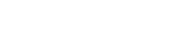# math.sqrt

`FLOATmath.sqrtFLOATx`

Available inall subroutines.

Computes the square root of its argument `x`.

## Parameters

`x` - Floating point value.

## Return Value

Upon successful completion, this function returns the square root of `x`.

If `x` is `math.NAN`, a NaN will be returned.

If `x` is ±0 or `math.POS_INFINITY`, `x` will be returned.

If `x` is a finite value < -0 or `math.NEG_INFINITY`, a domain error occurs and a NaN will be returned.

## Errors

If the `x` argument is < -0 or `math.NEG_INFINITY`, then `fastly.error` will be set to `EDOM`.

## Example

``````declare local var.fi FLOAT;declare local var.fo FLOAT;
set var.fi = 9.0;set var.fo = math.sqrt(var.fi);``````

BETA